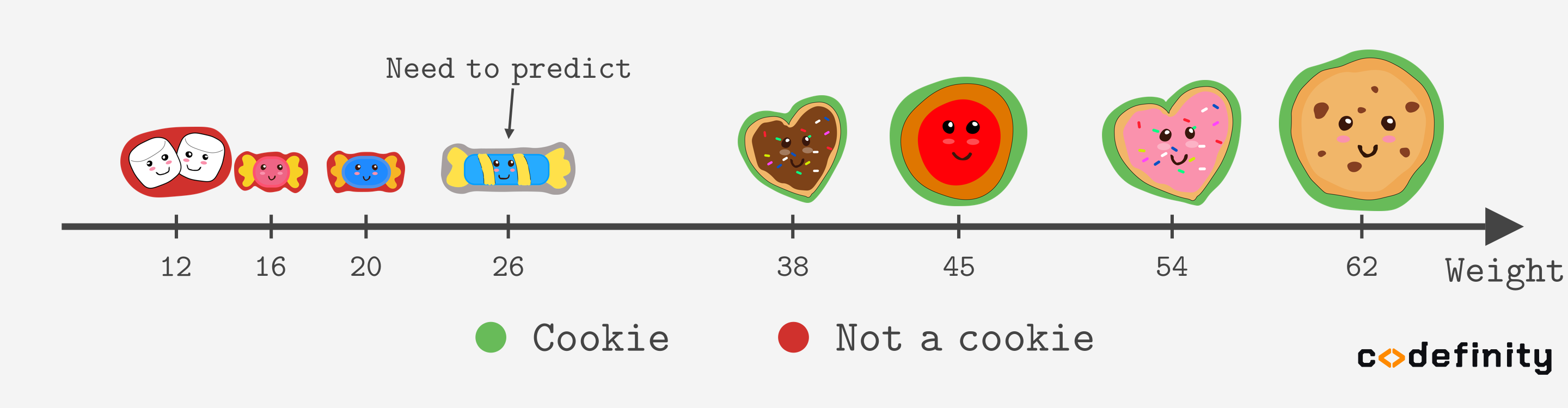Course Content

Classification with PythonWhat is k-NN

Let's start our classification adventure with the simplest task — binary classification with only one feature.
Suppose we want to classify sweets as cookies/not cookies based on their weight only.A simple way to predict the class of a new instance is to look at its closest neighbor. In our example, we must find a sweet that weighs most similarly to the new instance.

That is the idea behind k-Nearest Neighbors(k-NN) - we just look at the neighbors.
The k-NN algorithm assumes that similar things exist in close proximity. In other words, similar things are near each other.
k in the k-NN stands for the number of neighbors we consider when making a prediction.

In the example above, we considered only 1 neighbor, so it was 1-Nearest Neighbor. But usually, k is set to a bigger number. Looking only at one neighbor can be unreliable. Consider the example:

If k(number of neighbors) is greater than one, we choose the most frequent class in the neighborhood as a prediction.

Here is an example of predicting two new instances with k=3.

As you can see, changing the k may cause different predictions. We will talk about choosing the best k in the later chapters.
You may have wondered, what if an identic number of classes exists in the neighborhood? For example, if k=2 and the neighbors are different classes. In practice, the value of k is usually larger, and ties rarely happen. The Scikit-learn implementation does not even handle this case separately. It just chooses based on the order.

Everything was clear?

Section 1. Chapter 2